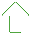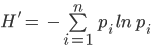#### Stand Complexity

Latest Score:

4.7/5

in 2019

Weight: 8%score trend is up over timeStand complexity is the proportion of trees in a set of diameter classes. Forest stands can be composed of trees in a variety of sizes, and stands with greater complexity in tree sizes are often more resilient to disturbances and other stressors, and more productive1. Complex stands can also provide more varied habitat for wildlife and sustain higher biological diversity. Here, stand complexity is assessed using the Shannon-Weiner Index, using the proportion of trees per 5" diameter size class. For a high score, the forest would have to have an equal number of trees in each size class represented.

1Buongiorno, J., Dahir, S., Lu, H.C. and Lin, C.R., 1994. Tree size diversity and economic returns in uneven-aged forest stands. Forest Science, 40(1), pp.83-103.

-- Expert interpretation for Stand Complexity is not available--

The score is calculated using a target value and the historical range of the the entire long-term dataset. The higher the score, the closer this year's value is to the target.

Once the score is computed for each year, the trend in scores over time is calculated. If the trend is significantly positive or negative, the long-term trend is marked as increasing or decreasing respectively.

Component Description
Scored as

Distance between minimum and maximum (scaled 1-5)

Target value

Data maximum + 10% of the range

Directionality of scores

Higher values in the data are better

Minimum value used in scoring

Data minimum -10% of the range

Maximum value used in scoring

Data maximum + 10% of the range

Using the USFS Forest Inventory and Analysis Program population estimate data on Phase 2 plots accessed via the FIA Datamart1, we computed tree size class diversity using a Shannon-Weiner Diversity Index calculation (see equation below). The first available data year was 1997. We divided all sampled trees into 5 inch classes and tallied the total proportion of each size class measured in the plots. To compute the annual score, we used FIA’s plot evaluation group panels to create population estimates for each year. The target for this dataset was set as the maximum value plus 10% of the range. The target was set as the upper scoring bounds (dataset maximum plus 10% of the range), and the current year is scored for where it falls between the lower scoring bounds (dataset minimum minus 10% of the range) and the target, scaled to be between 1 and 5.

Shannon-Weiner Index (H’)Where pi is the proportion of trees in the ith size class

1 USDA Forest Service. 2019. FIA Data Mart. Available at: https://apps.fs.usda.gov/fia/datamart/datamart.html